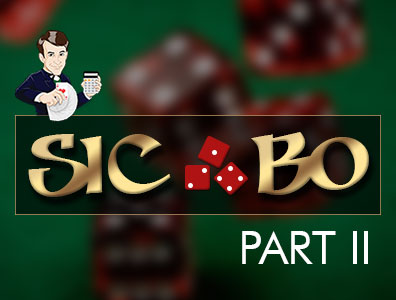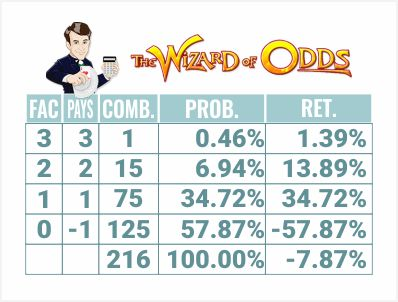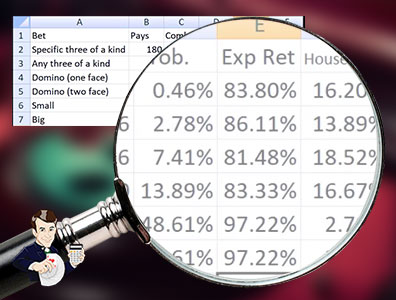## Wizard Recommends

Last Updated: October 3, 2018

# Sic Bo - Best Bets and Worst Bets | Video

Part 1

Hi, guys.

This is part two in my two video series on Sic Bo. In part one I explained how to calculate the probability of any given total in three dice, as well as the probability of throwing any given particular die face zero to three times in Sic Bo. Both of these are very fundamental bets in the game.In this part two:

I'm going to assume we already know the knowledge that we acquired in part one. We will see what are the best bets in Sic Bo, what are the worst and what kind of value this game offers to the player?

Let's jump right in, let's jump right in, shall we?

There are a whole bunch of bets in Sic Bo to talk about. So let's begin with the bets on any given total from 4 to 17. There is one bet for each of the numbers in this range. I already put down what each of these bets pay. This is on a "to 1" basis, meaning the player keeps his original bet back if he wins. This was taken from a Sic Bo game in Atlantic City.

I believe all the Sic Bo tables in Atlantic City follow the same rules as they do in most games. Casinos in other parts of the world may be more generous, maybe more stingy.Atlantic City is a good example

In part one of this series, I showed you how to calculate the number of combinations as well as the probability of any given total from 3 to 18. So I would refer you to go back and watch that video if you don't understand where these numbers came from or you can just take them on faith.

The expected return is what percentage of a bet the player can expect to get back on average. If it's a simple win or lose bet with only one way to win, this is really easy.

You take the probability and multiply it what the player gets back if he wins. I'm going to add one to these pays, because if the player wins not only does he get the winnings but he keeps his original bet.

For example: the bet on a total of four pays 60 to 1. The probability of winning is 1.39%. The expected return is 1.39% times 61.

We can simply copy and paste this formula down for all these different total bets. We can see the expected return ranges from- it looks like a low of 81.02% up to a high of 90.28%.

What is the difference between 100% and this expected return?

Well, that's called the "house edge" or the "house advantage." So the expected return plus the house advantage always equals 1 or 100%. To get the house advantage I just subtract- I just take one minus the expected return.

For example:

The house advantage on the bet on a total of four is 15.28%. It looks like the least it gets is 9.72% on the bets on 7 and 14. It gets as high as 18.98%. Ouch, I think that's on a total of 9 and 12.

Next…

…let's talk about the six bets on any particular die face. The way these bets work is you choose one of the faces from one to six, and if you've chosen- a face appears three times you win 3 to 1, twice 2 to 1, once 1 to 1. If it doesn't appear at all, you lose. Very simple. One, two, three.In part one of this series…

…I showed you how to calculate the number of ways your chosen face could appear zero to three times, which appears right here. If you don't understand where these numbers come from, I would invite you to go back and watch part one.

The way I like to analyze bets that have more than one possible outcome like this is to take the probability for all possible events of the probability times what it pays. I can just copy that down for all possible outcomes.

What this shows me is that for the player's bet:

He can expect to win 1.39% of it from the 3 to 1 win, 13.89% for the 2 to 1 win and so on. If we take the sum of all this, we get -7.87%. That means the player can expect to lose 7.87% of all the money he bets on this bet, negative meaning the player can expect to lose as he can expect to happen in almost any bet in the casino, especially in Atlantic City.

Another way

…we might express that is that the house advantage is 7.87%. I forgot to mention that in Australia on this bet if your chosen face appears three times, it doesn't pay 3 to 1, it pays 12 to 1. So that lowers the house advantage down to 3.7%. So, hooray for Australia.

Moving right along

Let's talk about four of the other types of bets you would find in any Sic Bo game. One of them is on a Specific three of a kind. For example, one, one, one, two, two, two, three, three, three. Each of these pays 180 to 1 in Atlantic City.

There is obviously only one way to win each of them. So, the probability is the number of combinations divided by the total possible. 216, six to the third power. As I showed you with the total bets, the expected return is a probability of winning times what it pays plus one. Again we add one because you get your original bet back if you win. The house advantage is simply one minus the expected return or what the casino can expect to keep.

This shows that on the specific three of a kind bets the probability of winning is 0.46%. The player can expect to get back 83.8% of his bet and give 16.2% to the casino.

Another bet is on Any three of a kind

This obviously wins on any three of a kind. You don't need to pick a specific one. However, the win is only 30 to 1, because there are six ways to win, one for each six of a kind. I mean, three of a kind.

The probability of winning is 2.78%. The house expected return goes up to 86.11% and the house advantage is 13.89%. These next two type of bets I to this day don't know the term for them.

I call them the "Domino bets," because the layout of on the table to bet these things looks like a Domino. For the parts of the layout that look like a 1:1 Domino, 2:2 Domino, 3:3 Domino. In other words, the same face twice. The way to win that is in between the three dice whatever that chosen number is must appear at least twice.For example:

If you bet on five five, then the only roles that would cause that to win would be five five one, five five two, five five three, five five four, five five five and five five six. Of course, in any order.

This bet, what I call a "one-face Domino," pays 10 to 1. The number of ways you can win it is if- let's say that you bet the five five, that third die, if it's different can be one of the other five faces. So we take a five there. The other die can be any one of the three dice so we multiply that by three.

For those types of wins there are 15 combinations. We also have to add one, because if you got three of that face you would win as well. The five five bet would win on a five five five.

We can copy and paste these cells down and see that the probability of winning is 7.41%, expected return 81.48% and the house advantage of 18.52%, ouch. Next, let's talk about what I call the "two-faced Domino bets." This might look on the layout of the table like three five, four six, one two.

In order to win:

Both of those faces must appear at least once between the three dice. For example, if you bet on three five, then the ways to win that would be one three five, two three five, three three five, four three five, five three five, or six three five.

Notice that in all those combinations…

…the three and the five appear at least once. Of course, it doesn't make any difference what order the dice come in. This bet pays 5 to 1.

There are two different types of ways you could win this bet. Let's say that you pick four six, for example. That third die could be something different. In this example, the one, the two, the three or the five.

If the third face that you didn't pick is different, it could be one of four different things or four different faces left. Between those three different faces, say three four five, they can be ordered in six different ways, three factorial.

The number of ways you can win

…where all three dice are different is 24. You can also win where one of them appears twice. For example, if you bet four six, you would win on four four six or four six six. Either one of them could appear twice. There's two different faces that could appear twice. We'll put it two. The number of ways you can arrange a pair and a singleton between three dice is three, because the singleton could be any one of the three dice. So, two times three.

The total number of combinations is 30

The probability of winning is 30 divided by 216 or 13.89%. Copy and paste these formulas down. Expected return two of 83.33% and the casino keeps 16.67%. Notice the high house advantage at least 13% on all these bets.

Under the Atlantic City pay table where this is what they pay, I highly recommend staying away from all of them. Next, let's talk about two of the most popular bets, the small and the big.

The small wins on a total of 3 to 10, except if that total is a three of a kind. I really should say 4 to 10, because of the total were three that can only be composed with a one one one, which is a three of a kind.

From the earlier part of this video…

…where I go over the totals, we see that the number of combinations for a total of 4 to 10 is 107, but we have to subtract 2 for that because the two two two and the three three three lose.

The two two two is a total of six and the three three three is a total of nine, both of which are under 10. We don't need to subtract for the four four four, five five five or six six six, because they would lose anyway.

There are 105 ways to win out of the 216. Copying and pasting the same formulas below we see that the probability of winning is 48.61%, expected return to the player of 97.22% and a house advantage of 2.87%, which compared to these other bets looks fantastic.

The big bet

…is basically the flip side of that. It wins on a total of 11 to 17. Again, except for the three of a kinds. The odds are exactly the same as on the small bet.

There's two things that I hope you've learned from this video:

One is that, if you must play Sic Bo, stick to the small and the big bets only. The house advantage on everything else is much, much higher. More importantly I hope that you learned a little bit of something about math and maybe using Excel.

There are some other bets in Sic Bo, but I think this has run long enough. Everything I talked about are all the major bets. I can't think of anything else to say, so thank you for watching.